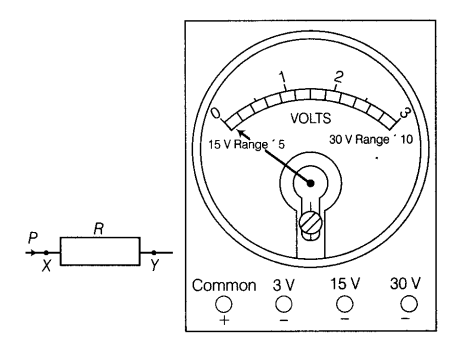# The scale of the voltmeter used in the experiment is shown as below:

The scale of the voltmeter used in the experiment is shown as below:(i) Why is the common terminal marked with a positive sign?
(ii) Explain your connection from X to the voltmeter terminal.

(i) It is to indicate that this terminal of the voltmeter should be connected to the positive (+) terminal of the battery, when the voltmeter is to be connected in an electric current.
(ii) As, the current is entering the resistor through the terminal X, it means that this terminal is connected to the positive terminal of the battery and it is at a high potential. So, while connecting a voltmeter across the resistor, the positive terminal of the voltmeter is connected to the terminal X of the resistor.